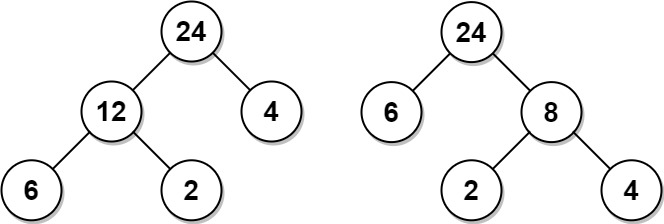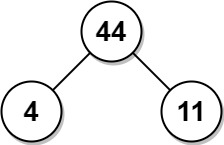1130. Minimum Cost Tree From Leaf Values
Medium
3.9K
258

Given an array `arr` of positive integers, consider all binary trees such that:

• Each node has either `0` or `2` children;
• The values of `arr` correspond to the values of each leaf in an in-order traversal of the tree.
• The value of each non-leaf node is equal to the product of the largest leaf value in its left and right subtree, respectively.

Among all possible binary trees considered, return the smallest possible sum of the values of each non-leaf node. It is guaranteed this sum fits into a 32-bit integer.

A node is a leaf if and only if it has zero children.

Example 1:```Input: arr = [6,2,4]
Output: 32
Explanation: There are two possible trees shown.
The first has a non-leaf node sum 36, and the second has non-leaf node sum 32.
```

Example 2:```Input: arr = [4,11]
Output: 44
```

Constraints:

• `2 <= arr.length <= 40`
• `1 <= arr[i] <= 15`
• It is guaranteed that the answer fits into a 32-bit signed integer (i.e., it is less than 231).
Accepted
85.8K
Submissions
125.8K
Acceptance Rate
68.2%

Seen this question in a real interview before?
1/4
Yes
No

Discussion (0)

Related Topics